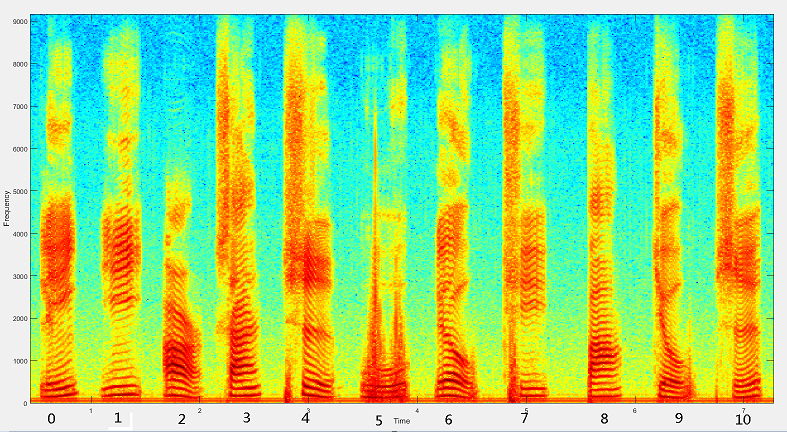# 还是不会VAD？三分钟看懂语音激活检测方法

(在苹果系统下，如果文章中的图片不能正常显示，请升级Safari浏览器到最新版本，或者使用Chrome、Firefox浏览器打开。)

VAD有很多种特征提取方法，一种最简单直接的是：通过短时能量(short time energy, STE)和短时过零率(zero cross counter, ZCC) 来测定，即基于能量的特征。短时能量就是一帧语音信号的能量，过零率就是一帧语音的时域信号穿过0（时间轴）的次数。一般来说，精确度高的VAD会提取基于能量的特征、频域特征、倒谱特征、谐波特征、长时信息特征等多个特征进行判断。最后我们再根据阈值进行比较，或者使用统计的方法和机器学习的方法，得出是语音信号还是非语音信号的结论。

## 1 特征方法

### 1.1 基于能量的特征一段语音信号的频谱图示例

## 2 判别方法

### 2.1 阈值法

$$\eta= \left\{\begin{matrix} \eta_0 & E\le E_0 \\ \frac{\eta_0-\eta_1}{E_0 -E_1} E + \eta_0 – \frac{\eta_0-\eta_1}{1-E_1/E_0} & E_0 < E < E_1 \\ \eta_1 & E \ge E_1 \end{matrix}\right. \tag {1}$$

$$\hat \eta=\alpha \eta+(1-\alpha)\eta_{new} \tag{2}$$

### 2.2 统计模型方法

$$p(X_k|H_N)=\Pi_{i=1}^L \frac{1}{\pi \lambda_{N,i}}\exp(-\frac{|X_k,i|^2}{\lambda_{N,i}}) \tag{3}$$
$$p(X_k|H_S)=\Pi_{i=1}^L\frac{1}{\pi(\lambda_{N,i}+\lambda_{S,i})}\exp(-\frac{|X_{k,i}|^2}{\lambda_{N,i}+\lambda_{S,i}}) \tag{4}$$

$$\Lambda_i=\frac{p(X_{k,i}|H_S)}{p(X_{k,i}|H_N)}\tag{5}$$
$$\log \Lambda=\frac{1}{L}\sum_{i=1}^L \log \Lambda_i,if \log \Lambda \ge \eta,then, H_S,else H_N \tag {6}$$

## 参考资料 Refference

1. 语音检测(VAD)原理和实例, https://shichaog1.gitbooks.io/hand-book-of-speech-enhancement-and-recognition/content/chapter7.html
2. 关于语音端点检测（Voice Activity Detection，VAD）的一些汇总 https://blog.csdn.net/baienguon/article/details/80539296
3. RONAO C A, CHO S B. Human activity recognition using smartphone sensors with two-stage continuous hidden Markov models[C]//Proceedings of the 201410th International Conference on Natural Computation. Piscataway, NJ:IEEE, 2014:681-686.
4. 李强, 陈浩, 陈丁当. 基于隐马尔可夫模型的语音激活检测算法[J]. 计算机应用, 2016, 36(11): 3212-3216.DOI: 10.11772/j.issn.1001-9081.2016.11.3212.
LI Qiang, CHEN Hao, CHEN Dingdang. Voice activity detection algorithm based on hidden Markov model[J]. Journal of Computer Applications, 2016, 36(11): 3212-3216. DOI: 10.11772/j.issn.1001-9081.2016.11.3212.
 版权声明本博客的文章除特别说明外均为原创，本人版权所有。欢迎转载，转载请注明作者及来源链接，谢谢。本文地址: https://blog.ailemon.me/2021/02/18/introduction-to-vad-theory/ All articles are under Attribution-NonCommercial-ShareAlike 4.0